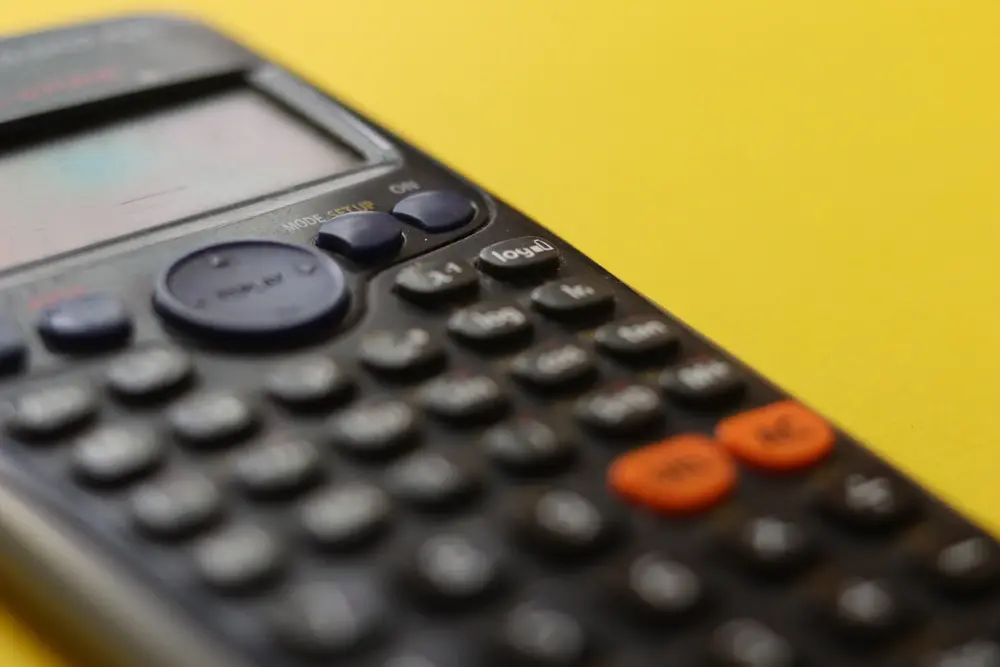# Errors

#### Absolute Errors

• Since no measurement is exact, there will always be the possibility of an error. Numbers are often rounded off to a certain number of significant figures or decimal places, and in such instances an absolute error can be caluclated.
• If the number 1200 is given to the nearest 100, for example, then the number can be anything between 1150 and 1250. It can therefore be written as 1200 ± 50. In this instance, the absolute error is 50.
• In general, if a number is a ± b, then the absolute error is b .

#### Relative Errors

• The absolute error doesn't really tell us much about how big the error really is. For example, an absolute error of 1000 is very big if the number we are talking about is 3000, but it is small if we are talking about 100 000 000 000. The relative error incorporates the number that we are talking about.
• If a number is a ± b, then the relative error is b/a
• Example
• If 200 is correct to 2 significant figures, what is the relative error?
• This can be written as 200 ± 5, since the highest number it could be is 205 and the lowest is 195. The relative error, therefore, is 5/200 = 1/40 = 0.025 .

#### Percentage Error

• Percentage error = relative error × 100
Numerical methods